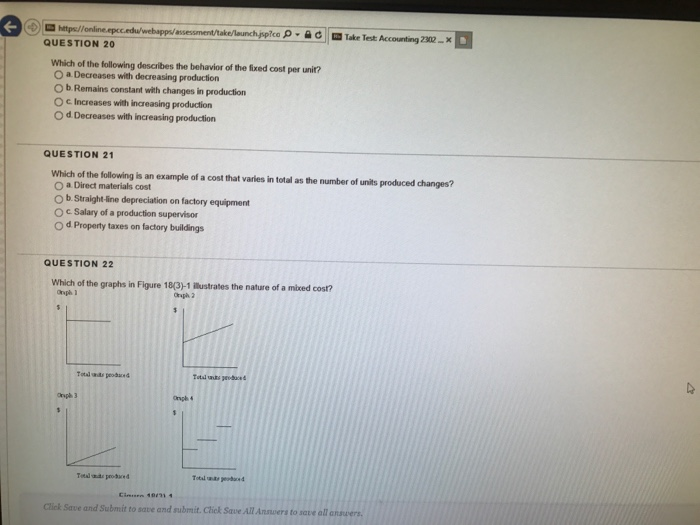# Which of the following describes the behavior of the fixed cost per unit?Which of the following describes the behavior of the fixed cost per unit? a. Decreases with decreasing production b. Remains constant with changes in production c. Increases with increasing production d. Decreases with increasing production Which of the following is an example of a cost that varies in total as the number of units produced changes? a. Direct materials cost b. Straight-line depreciation on factory equipment c. Salary of a production supervisor d. Properly taxes on factory buildings Which of the graphs in Figure 18(3)-1 illustration the nature of a mixed cost?

Ans 20 d. Decreases with increasing production
Fixed cost remains same at all the level of output as we
increase output fixed cost per unit starts decreasing.
Fixed cost per unit = fixed cost ÷ no of units produced
Ans 21 a. Direct Material Cost
Director material cost varies in totality as the no of units
produced changes. There is direct relationship between direct
material cost and no of units produced.
All other options are incorrect
Ans 22. Graph 2
Mixed cost are those cost which are fixed as well as variable.
So, initially, there is component of fixed costs due to which graph
starts from little upward. After that point, it start increasing
due to variable component.